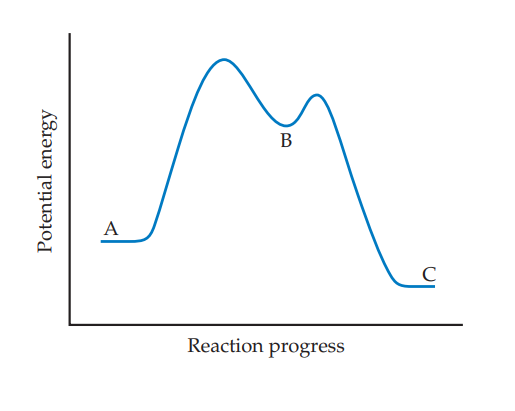# ?Based on the following reaction profile, how many intermediates are formed in the reaction $$\mathrm{A} \longrightarrow \mathrm{C}$$? How many transit

Chapter 14, Problem 14.13

#### (choose chapter or problem)

Based on the following reaction profile, how many intermediates are formed in the reaction $$\mathrm{A} \longrightarrow \mathrm{C}$$? How many transition states are there? Which step, $$\mathrm{A} \longrightarrow \mathrm{B}$$ or $$\mathrm{B} \longrightarrow \mathrm{C}$$, is the faster? For the reaction $$\mathrm{A} \longrightarrow \mathrm{C}$$, is $$\Delta E$$ positive, negative, or zero? [Section 14.6]Text Transcription:

A \longrightarrow C

A \longrightarrow B

B \longrightarrow C

A \longrightarrow C

\Delta E

Unfortunately, we don't have that question answered yet. But you can get it answered in just 5 hours by Logging in or Becoming a subscriber.